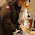## Saturday, July 20, 2013

### Decisions from posterior distributions: Tail probability or highest density interval?

How should we decide whether a parameter's posterior distribution "rejects" a particular value such as zero? Should we consider the percentage of the distribution above/below the value? Should we consider the relation of the highest density interval (HDI) to the value? Here are some examples to explain why I think it makes more sense to use the HDI.

Here are the two decision rules being compared. First, the tail-probability decision rule: If there is less than 2.5% of the distribution on either side of the value, then reject the value. This is tantamount to using a 95% equal-tailed credibility interval: Values outside the 95% equal-tailed credibility interval are "rejected." Second, the HDI decision rule: Values outside the 95% HDI are "rejected." (Of course, I like to enhance the decision rule with a ROPE, to allow acceptance decisions and to provide a buffer against false alarms -- but that's a separate discusssion.)

The two histograms below represent the MCMC results for two hypothetical parameters.
The upper panel shows a posterior distribution for which the parameter value of zero falls will within the 95% HDI, but 2.0% (<2.5%) of the distribution falls below the value zero. Do we "reject" zero or not? I think it would be wrong to reject zero, because the probability density (i.e., credibility) of zero is high. Zero is clearly among the most credible values of the parameter, even though less than 2.5% of the distribution falls below zero.

The lower panel shows a posterior distribution for which the parameter value of zero falls well outside the 95% HDI (thus, even with of a modest ROPE, e.g. from -1 to +1, zero would still fall outside the 95% HDI), but a full 3.0% (>2.5%) of the distribution falls below zero. Do we "reject" zero or not? If we use a tail-probability decision rule, we do not reject zero. But clearly zero is not among the most credible values of the parameter, in that zero has low probability density.

Proponents of using equal-tailed credibility intervals will argue that percentiles of distributions are invariant under transformations of the parameter, but HDIs are not. True enough, but I think that most parameters are specifically scaled to be meaningful, and we want to know about credibility (probability density) on the meaningful scale, not on a meaningless transformed scale. But I am not saying that one decision rule is "correct" and the other is "wrong." The decision rules are merely rules with differing interpretations and characteristics; I am showing examples that convince me that a more useful, intuitive rule is using the HDI not the equal-tailed interval.

Then why do the plots in DBDA (like those above) bother to display the percentage of the distribution below/above the comparison value? Primarily merely as an additional descriptive statistic, but also to inform those who wish to think about tail probabilities for a decision rule that I eschew.

For another example, see this previous post.

1.The question of whether to prefer a quantile-based interval or an HDI can be clarified by taking a statistical decision theory perspective. Consider two loss functions, both of which consist of a penalty proportional to the interval width plus another term that penalizes lack of coverage of the true value. For the first loss function, the lack-of-coverage penalty is the 0-1 loss; for the second loss function, the lack-of-coverage penalty is proportional to the distance by which the interval fails to cover the true value (if any). It turns out that minimizing posterior expected loss leads to the HDI under the first loss function and to a quantile-based interval under the second loss function.

2.Dear Prof. Krushke, I'm working with ch 22 of DBDA to analyse contingency tables. I'm curious to know where to look for a measure of effect size, both for the whole table and for individual interactions. Any tips you have would be much appreciated!
Thanks, Ben

3.Thanks for your interest in the book.

My short answer is that I don’t have an adequate answer. There are many relevant measures of effect size in the frequentist literature, of course, but we seek something for hierarchical Bayesian log-linear models. A measure of effect size is supposed to be an aggregated difference (i.e., the “effect”) standardized relative to some indicator of variance or noise in the data. Moreover, in a Bayesian setting, the effect size has a posterior distribution; it’s not just a point estimate. An example of posterior effect size for a difference between groups is given here: http://www.indiana.edu/~kruschke/BEST/
One possibility for describing effect size in hierarchical Bayesian ANOVA-style models (including log-linear models) is a ratio of estimated variance parameters. For example, the main effect of factor A might have an “effect size” given by sigma_A divided by sigma_y, where sigma_A is the scale parameter of the distribution of the factor-A deflections, and sigma_y is the scale parameter of the within-cell noise distribution. But that’s just off the top of my head.
Thanks again,
--John

4.I am trying to get a feel for peoples preference on equal-tailed -vs- highest density. So, I made a google-form mini survey here:

Currently, with only very few respondents, the preference appears to be about balanced ETI vs HDI.

For me personally the invariance to reparameterization of the equal tailed interval is very appealing. Lets say an astronomer does sine regression to characterize the dominant oscillation in a time series. I would expect consistent intervals regardless on whether a period or a frequency was used as the parameter. Often I am interested in the sign of a parameter, then i'd like to know the relative odds of positive vs negative (or simply the probability of negative as you show in green in your plots).

5.6.